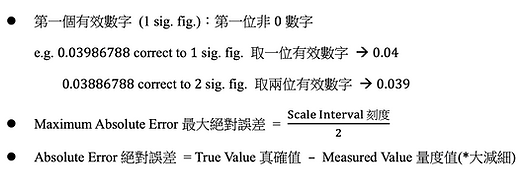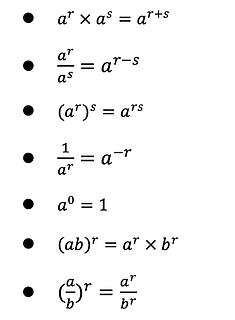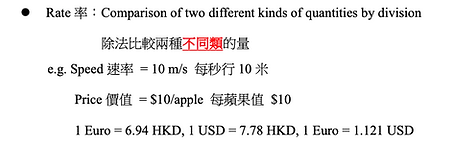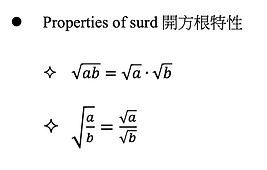top of page

## F.2 Formula 中二數學公式

table of content

Beyond Math《F.2 Formula 中二數學 數學公式》 整合了 中二數學 課程重點，希望幫助 數學補習 同學查閱各個數學課題公式，明白處理題目的方法及技巧，節省課餘温習時間。立即Like我地Facebook 同 follow我們Instagram獲取最新免費的中學 數學補習 特別資訊。

# 2022年 數學補習 同學 要留意的 中二數學 數學公式

Approximation and ErrorApproximation and Error

Manipulation of PolynomialsManipultion

Identities 恆等式Identities
Factorization of Polynomials

Factorization of PolynomialsAlgebraic Fraction

Algebraic Fraction and FormulaeLinear Equation

Linear Equations of Two Unknown

一元二次方程StatisticsRate & Ratio

Rate and Ratio 率與比Angles in Tri

Angles in Triangles and PolygonsDeductive Geo

Deductive Geometry 演繹幾何Pyth

Pythagoras’ Theorem & Square RootTri

Trigonometric Ratios 三角比Area

Area and Volume 面積和體積## Beyond Math 教學理念

Beyond Math 透過系統化，為每個學生度身訂造目標同進度。在課堂中，分topic 去溫，先從較容易的topic 開始，透過Past Paper 同唔同題目做練習，逐個topic 去go through，最後一定可以應付DSE數學。

Past Paper 及bottom of page